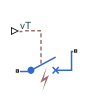# Circuit Breaker (with arc)

Single-pole single-throw circuit breaker with Mayr arc representation

• Library:
• Simscape / Electrical / Switches & Breakers

•## Description

The Circuit Breaker (with arc) block represents a single-phase circuit breaker with Mayr arc representation controlled by an external control signal vT. If vT is less than the threshold, then the breaker is closed. If vT is greater than or equal to the threshold, then the breaker opens with an arc during the current interruption. The external signal can open and close the breaker repeatedly.

The table shows how the external signal vT controls the block behavior.

ConditionBlock Behavior
vT < ThresholdThe circuit breaker is closed. Port 1 is connected to port 2.
vT ThresholdThe circuit breaker is either opening or open. Port 1 is connected to port 2 via a nonlinear conductance.

The Circuit Breaker (with arc) block has a higher computational overhead than the Circuit Breaker block. If the fidelity of the representation of arc current or voltage is your overriding requirement, use the Circuit Breaker (with arc) block and use a global Simulink® variable-step solver. Otherwise, use the Circuit Breaker block.

### Mayr Arc Model Equations

The defining equations for the breaker are

`$x=\mathrm{ln}\left(g\right)$`

and

`$i=gv,$`

where:

• g is the arc conductance.

• x is an internal state variable.

• v is the voltage across the breaker.

• i is the current through the breaker.

When the breaker is closed,

`$\frac{dx}{dt}=0.$`

When the breaker is opening or open,

`$\frac{dx}{dt}=\frac{1}{\tau }\left(\frac{g{v}^{2}}{P}-1\right),$`

where:

• τ is the arc time constant.

• P is the cooling power.

## Ports

Refer to the figure for port locations.### Input

expand all

Scalar physical signal control port.

#### Dependencies

To enable this port, set Modeling option to `PS control port`.

### Conserving

expand all

Electrical conserving port.

Electrical conserving port.

Positive electrical conserving port.

#### Dependencies

To enable this port, set Modeling option to `Electrical control port`.

Negative electrical conserving port.

#### Dependencies

To enable this port, set Modeling option to `Electrical control port`.

## Parameters

expand all

Whether to specify physical or electrical control port for the switch gate.

Time constant for the Mayr arc..

Cooling power.

Conductance between ports 1 and 2 when the breaker is closed.

Threshold voltage for the control port vT. The block uses the threshold voltage and the value of vT at the start of the simulation to determine whether the breaker is initially open or closed. When the voltage rises above the threshold, the breaker opens. When the control port voltage falls below the threshold, the breaker closes.

 Schavemaker, P. H., and L. Van der Sluis. “The Arc Model Blockset.” Proceedings of the Second IASTED International Conference POWER AND ENERGY SYSTEMS (EuroPES). Crete, Greece, June 25-28, 2002, pp. 644-648.